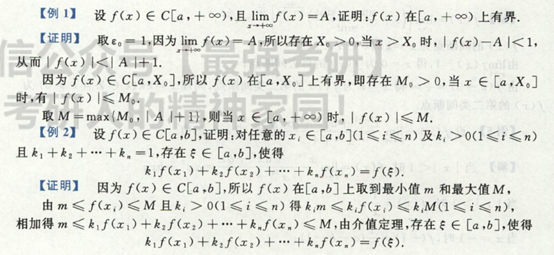• 视频21四、连续函数闭区间上的性质函数在区间I上的最大值和最小值定义设函数f(x) 在区间I 上有定义如果x0 属于I，使得任意 x 属于 If(x0) &lt;= f(x) (或 f(x0) &gt;= f(x) ) 则称f(x0)是f(x)在区间I上的...
视频21四、连续函数在闭区间上的性质函数在区间I上的最大值和最小值定义设函数f(x) 在区间I 上有定义如果x0 属于I，使得任意 x 属于 If(x0) <= f(x) (或 f(x0) >= f(x) ) 则称f(x0)是f(x)在区间I上的最小值（f(x0)是区间I上的最大值）记为minf(x) = f(x0)(x属于区间I)maxf(x) = f(x0)(x属于区间I)1 最大最小值定理， 闭区间上的连续函数，在该区间上一定有最大值和最小值解释注意：“闭区间” 、“连续”  两个条件不可少例1 y=1/x 在 （0，1）连续， 它即无最大值、也无最小值例2 不连续的函数2 有界性定理：闭区间上连续的函数，在该区间上它一定有界。证明：设f(x) 在闭区间[a,b]上是连续的,由性质1可知，在闭区间[a,b]内一定存在最大值和最小值 使得m<=f(x)<=M,x属于[a,b],f（x）在闭区间[a,b]既有上界也有下界，从而推出f(x) 在区间【a,b】上有界3 零值点定理使得函数f(x)的函数值等于0的点x0, 即f(x0)=0,称x0为f(x)的零值点。设f(x)在[a,b]上是连续的， 且f(a)与f（b）的积小于0,则至少存在一点 ξ 使得 f(ξ) = 04 介值定理设f(x) 属于[a,b],且f(a) = A,f(b) = B , A <> B, 则对于数C介于A与B之间， 则至少存在一点ξ使得f(ξ) = C,证明：使用3 证明 4推论：设f(x)在闭区间[a,b]上连续，令 m= minf(x),M = maxf(x) (x属于[a,b])而数μ，m < μ < M,则比存在一点 ξ 使得f(ξ) = μ 例1 f(ξ ) = [f(x1) + f(x2) + .. + f(xn) ] / n由推论证习题：2-6 ， 9（2）（3）（4）（5）（7），11，12
展开全文高等数学
• 文章目录闭区间连续函数的性质1.有界性定理2.最值定理3.零点存在定理4.介值定理5.一致连续定理(Cantor定理) 闭区间连续函数的性质 闭区间上的连续函数具有许多特殊的性质，在开区间上不一定具有。 1.有界性定理 命题...
文章目录闭区间连续函数的性质1.有界性定理2.最值定理3.零点存在定理4.介值定理5.一致连续定理(Cantor定理)
闭区间连续函数的性质
闭区间上的连续函数具有许多特殊的性质，在开区间上不一定具有。
1.有界性定理
命题：若函数$f(x)$在闭区间$[a,b]$上连续，则它在$[a,b]$上有界。
证明：用反证法。
如果$f(x)$在$[a,b]$上无界，则将$[a,b]$等分为两个小区间$[a,\dfrac{a+b}2],[\dfrac{a+b}{2},b]$，函数$f(x)$至少在其中一个小区间上无界，记作$[a_1,b_1]$；继续等分，函数依然至少在一个半区间上无界，记作$[a_2,b_2]$；依此进行，得到一个闭区间套$\{[a_n,b_n]\}$，这样就存在一个唯一的实数$\xi$属于所有区间$[a_n,b_n]$，且
$\xi=\lim_{n\to \infty}a_n=\lim_{n\to \infty}b_n.$
由于$f(\xi)$在$[a,b]$上连续，$f(x)$在$\xi$处连续，即$f(\xi)=\lim\limits_{x\to \xi}f(x)$存在，所以存在一个$\delta$，使得$f(x)$在$U^\circ(\xi,\delta)$有界（这是函数极限的有界性），结合函数的连续性，对于一切$x\in U(\xi,\delta)\cap [a,b]$存在
$|f(x)|\le M.$
由于闭区间套的长度趋近于0，所以一定存在一个$N$，使得$n>N$时，$b_n-a_n<\delta/2$，这就意味着
$[a_n,b_n]\sub U(\xi ,\delta),$
然而$f(x)$在$[a_n,b_n]$上无界，在$U(\xi,\delta)$上有界，这显然是矛盾的。
综上所述，闭区间上的连续函数有界。
开区间上连续函数无界的例子：$f(x)=\dfrac 1x$在$(0,1)$上无界。
2.最值定理
命题：若$f(x)$在闭区间$[a,b]$上连续，则它在$[a,b]$上必能取到最大值和最小值，即存在$\xi,\eta\in [a,b]$，对于一切$x\in [a,b]$，有$f(\xi)\le f(x)\le f(\eta)$。
证明：可以先找比最值稍弱的值——确界，再通过确界向最值逼近。
由有界性定理得$f(x)$在$[a,b]$上有界，所以$f(x)$的值域$R_f$存在下确界$\alpha=\inf R_f$和上确界$\beta =\sup R_f$。要证明存在$\xi\in [a,b]$使得$f(\xi)=\alpha$。
根据下确界的定义，一方面$f(x)\ge \alpha$，另一方面$\forall \epsilon>0,\exist x,f(x)<\alpha+\epsilon$，于是取$\{\epsilon_n\}$趋近于$0$，不妨设$\epsilon_n=\dfrac 1n$，由此得到数列$\{x_n\}$，其中$\alpha\le f(x_n)<\alpha+\frac 1n$。
由于$\{x_n\}$是一个有界数列，所以存在收敛子列$\{x_{n_k}\}$，由于$\{x_n\}$的构造方式，有
$\alpha\le f(x_{n_k})<\alpha+\frac 1{n_k}<\alpha+\frac 1k.$
两边同时关于$k$取极限，有$f(x_{n_{k}})\to \alpha$。不妨设$x_{n_{k}}\to x'$，那么由函数的连续性，有
$f(x')=f(\lim_{k\to \infty}x_{n_k})=\lim_{k\to \infty}f(x_{n_k})=\alpha,$
这就证明了$\exist x',f(x')=\alpha=\inf R_f$，证明了$f(x)$在$[a,b]$上取得到最小值。
同理构造收敛子列，可以证明$f(x)$在$[a,b]$上取得到最大值。
3.零点存在定理
命题：若函数$f(x)$在闭区间$[a,b]$上连续，且$f(a)f(b)<0$，则一定存在$\xi\in (a,b)$使得$f(\xi)=0$。
证明：要证明存在这样的$\xi$，关键是将其构造出来。
不失一般性，设$f(a)<0,f(b)>0$，可以定义集合$V=\{x|f(x)<0,x\in [a,b]\}$，显然集合$V$有界非空（至少含有点$a$），所以必有上确界，设为$\xi=\sup V$，现在想要证明$\xi\in (a,b),f(\xi)=0$。
先证明$\xi\in (a,b)$，由于$f(a)<0$，所以存在一个$\delta_1$使得$\forall x\in [a,a+\delta_1]$，$f(x)<0$；由于$f(b)>0$，所以存在一个$\delta_2$使得$\forall x\in [b-\delta_2,b]$，$f(x)>0$，这样就有$a+\delta_1\le \xi\le b-\delta_1$，所以$\xi\in(a,b)$。
取$x_n\in V,x_n\to \xi$，因为$f(x_n)<0$，所以$f(\xi)=f(\lim\limits_{n\to \infty}x_n)=\lim\limits_{n\to \infty}f(x_n)\le 0$，下证明$f(\xi)<0$是不可能存在的。
若$f(\xi)<0$，则根据$f(x)$在$\xi$的连续性，存在一个$\delta$，使得$[\xi-\delta,\xi+\delta]$内的$x$满足$f(x)<0$，这就与$\xi$是$V$的上确界矛盾（因为$\xi+\delta>\xi$且$f(\xi+\delta)<0$），所以必定有$f(\xi)=0$。
4.介值定理
命题：如果函数$f(x)$在闭区间$[a,b]$上连续，则它一定能取到最大值$M=\max\{f(x)|x\in [a,b]\}$和最小值$m=\min\{f(x)|x\in [a,b]\}$之间的任何一个值。
证明：建立介值定理与零点存在定理的联系。
由最值定理，存在$\xi,\eta\in [a,b]$使得$f(\xi)=m,f(\eta)=M$，不妨假设$\xi<\eta$。
对于任意中间值$C$即$m，考察辅助函数
$g(x)=f(x)-C,$
显然$g(x)$在$[a,b]$上也是连续函数，且$g(\xi)=m-C<0,g(\eta)=M-C>0$，由零点存在定理，必定有$\zeta\in (\xi,\eta)$，使得
$g(\zeta)=0=f(\zeta)-C,\quad f(\zeta)=C.$
这就证明了介值定理。同时，介值定理表明了$f(x)$在$[a,b]$上的值域为$R_f=[m,M]$。
5.一致连续定理(Cantor定理)
一致连续性：设$f(x)$定义在区间$X$上，$\forall \varepsilon>0,\exist \delta(\varepsilon)>0$，只要$|x_1-x_2|<\delta(x_1,x_2\in X)$，就有$|f(x_1)-f(x_2)|<\varepsilon$，就称$f(x)$在区间$X$上一致连续。
一致连续的充要条件：$f(x)$在$X$上一致连续，等价于对任何点列$\{x_n'\},\{x_n''\}(x_n',x_n''\in X)$，有
$\lim_{n\to \infty}(x_n'-x_n'')=0\Rightarrow \lim_{n\to \infty}(f(x_n')-f(x_n''))=0.$
命题(Cantor)：若函数$f(x)$在闭区间$[a,b]$上连续，则它在$[a,b]$上一致连续。
证明：采用反证法。
如果$f(x)$在$[a,b]$上不是一致连续的，则存在$\varepsilon_0>0$与点列$\{x_n'\},\{x_n''\}$满足
$|x_n'-x_n''|<\frac 1n,\quad |f(x_n')-f(x_n'')|\ge\varepsilon_0.$
由致密性定理，存在收敛的子列$\{x_{n_k}'\}$使得$\lim\limits_{k\to \infty}x_{n_k}'=\xi,\xi\in[a,b]$，在点列$\{x_n''\}$中选取同样下标的点列$\{x_{n_k}''\}$，则$|x_{n}'-x_{n_k}''|<\frac 1{n_k}$，且$|f(x_{n_k}')-f(x_{n_k}'')|\ge \varepsilon_0$，且
$\lim_{k\to \infty}x_{n_k}''=\lim_{k\to \infty}(x_{n_k}''-x_{n_k}')+\lim_{k\to \infty}x_{n_k}'=\xi.$
由于函数$f(x)$在$\xi$连续，所以
$\lim_{k\to \infty}f(x_{n_k}')=\lim_{k\to \infty}f(x_{n_k}'')=f(\xi).$
于是
$\lim_{k\to \infty}[f(x_{n_k}')-f(x_{n_k}'')]=0,$
这与$f(x_{n_k}')-f(x_{n_k}'')\ge \varepsilon_0$矛盾，因此一定是一致连续的。
该定理在开区间上不成立，是因为$\xi$可能取到区间的边界。


展开全文• 回顾 什么叫函数闭区间连续？ 用图像表示 定理1：最值定理 若f(x)∈c[a,b],则在[a,b]上一定能够取到最小值m和...注解： 由定理1我们知道，函数闭区间连续，则函数闭区间上可以取到最小值和最大值，即函数
回顾
什么叫函数在闭区间上连续？用图像表示定理1：最值定理若f(x)∈c[a,b],则在[a,b]上一定能够取到最小值m和最大值M，即存在x1，x2属于[a,b]使得f(x1)=m，f(x2)=M
注解： 函数在闭区间上连续是能取到最小值和最大值的充分不必要条件，如图定理2：有界定理
若f(x)∈c[a,b]，则存在k,对任意的x∈[a,b]有|f(x)|≤k，即函数在闭区间上连续，则函数在闭区间上有界
注解： 由定理1我们知道，函数在闭区间上连续，则函数在闭区间上可以取到最小值和最大值，即函数有上下界，则函数在闭区间内有界
定理3：零点定理
零点：f(x)在x=a处的值为0，则x=a称为f(x)的零点
若f(x)∈c[a,b]，且f(a)×f(b)<0，则存在f(x0)=0（至少有一个）定理4：介值定理
啥叫介值呢？画个图f(x)∈c[a,b]，在[a,b]上有最小值m和最大值M
用英文解释一下：The value between m and M 翻译过来，介于闭区间上最小值和最大值的值都叫介值
介值定理的内容：任取t∈[m,M],至少有一个t0使得f(t0)=t，则t0∈[a,b]，即介于m和M之间的值，f(x)都能取到
小结
①f(x)∈c[a,b]，存在t∈(a,b)…命题为闭区间，证明开区间，首选零点定理
②f(x)∈c[a,b]，出现条件有函数值之和，或t∈[a,b]，首选介值定理
例题
例1例2总结
本篇内容为f(x)在闭区间上连续的四个定理，当命题中有证明开区间上点的时候，首选零点定理，当命题中证明闭区间上的点或出现函数值之和时，首选介值定理，使用介值定理的惯例——先取出最小值和最大值
截止到本篇，高等数学第一章《函数、极限、连续》的内容已经基本完结，为什么说基本完结呢？因为第一章的内容相对基础（虽然基础但绝对重要），在后续的学习中可能会不断补充相关的新的知识。
接下来我们进入第二章——《导数与微分》


展开全文• 1、 闭区间及无线区间上连续函数的性质
1、	闭区间及无线区间上连续函数的性质展开全文• 根据实数理论，分析了闭区间连续函数的映射特点，得到了一个反映其规律的结论。
• 一、有界性与最大最小值定理 二、零点定理与介值定理 2.1、零点定理 2.2、介值定理 2.2.1、推论
• 若f(x)f(x)f(x)在[a,b][a,b][a,b]上连续，则对f(x)f(x)f(x)在该区间内的最大值M和最小值N而言， 必然有ϵ,ξ∈[a,b]\epsilon,\xi\in[a,b]ϵ,ξ∈[a,b]使得： N=f(ϵ)≤f(x)≤f(ξ)=MN=f(\epsilon)≤f(x)≤f(\xi)=MN=...
• §1.11 闭区间连续函数的性质 如果函数在开区间内连续，在右端点左连续，在左端点右连续，那未函数就在闭区间上连续。 一、最大值与最小值定理 先介绍最大值与最小值概念： 对于区间上有定义的函数，如果有...高等数学
• 020:闭区间连续函数性质之零点定理、介值定理
• 定理1：最值定理 定理2：有界定理 定理3：零点定理 定理4：介值定理
• 设$[a,b]$是$\mathbf{R}$上的闭区间,且$f(x)$是$[a,b]$上的连续函数,则$f(x)$在$[a,b]$上一致连续. 证明:反证法.假设$f(x)$在$[a,b]$上不是一致连续的,则必定存在这么两个序列,其中$$a_1,a_2,\cdots,a_n,\cdots$$...
• 1. 问题引入——任意面积的大理石，是否可以用锯子以直线锯口...3. 最值定理（闭区间上的连续函数一定存在最大值和最小值；引理：闭区间上的连续函数一定有界） 4. 零值定理 5. 介值定理 ...
• 定理一：（有界性与最大值最小值定理） 在闭区间连续函数在该区间上有界且一定能取得它的最大值和最小值 注意：在开区间上/闭区间内有间断点时上述定理就不一定成立了 定理二：（零点定理）设函数F(x)在闭区间...
• 数分思维导图整理（二）实数基本定理及闭区间连续函数性质的证明 更多系列文章请见作者专栏，有问题或建议欢迎留言。
• 对于我而言，高数的概念多，证明难，前后知识的联系深，学习起来具有一定难度。故想通过思维导图结合教材与辅导资料来复盘课上内容，以便加深记忆，回顾总结。同时也帮助各位在学习这本书的同学一个纲要。...
• 转载于:https://www.cnblogs.com/ccczf/p/9678438.html
• 对于开区间，本身已经不包含两端点值，所以根本满足不了连续...在已经证得该函数在该闭区间连续，之后在两端点处，左极限等于左端点的函数值，右极限等于右端点的函数值，那么就可以说明函数在该闭区间连续。 举例python
• 高等数学（同济七版）经典必备课件PPT，第一章第十节！
• 熟悉缠中说禅的股民，对闭区间套定理一定不会陌生。闭区间套定理是实数集上序列收敛的基本定理，它的发现者是天才数学家康托尔（Cantor）。康托尔是现代公理化集合论的奠基者。与许多的天才理论一样，他的集合理论在...
• 即，在闭区间上，一个单元函数满足后者一定可以推出 其也满足 前面的系列性质。即，闭区间上，从后往前推可以，但从前往后推，未必。具体表现为： 可导一定连续，可导一定可积，可导一定有界。 连续一定可积，...
•数学
• F（x）在闭区间I连续，在I区间可导，导函数在区间I不一定连续 实例： matlab代码如下： %绘制区间[-1,0)上的函数图像 x=[-1:0.001:0]; %计算对应点处f(x)函数值 y=(-x).^(3/2).*sin(1./-x) %计算对应点处f...matlab 极限定理
• 连续函数的四则运算复合函数的运算法则例题推论（复合函数的连续性）例题闭区间连续函数的性质介值定理根的存在定理...